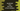# JavaScript program to find the lcm of two numbers## JavaScript program to find the LCM of two numbers:

In this post, we will learn how to find the LCM of two numbers in JavaScript. LCM is also known as the least common multiple or lowest common multiple. It is the smallest positive number that is divisible by both of these numbers.

For example, if the numbers are 5 and 6, the LCM is 30.

Multiples of 5 are 5, 10, 15, 20, 25, 30, 35, 40… and multiples of 6 are 6, 12, 18, 24, 30, 36, 42…. Here, 30 is the least number that is divisible by both 5 and 6.

### Method 1: By using a loop:

In this method, we will find the LCM by dividing the multipliers of the larger number by the smaller number. The LCM is the value that is divisible by the smaller number. Since it is a multiplier of the larger number, it is anyway divisible by the larger number.

Let’s take a look at the program:

``````let find_lcm = (first, second) => {
let larger_value = first > second ? first : second;
let smaller_value = first < second ? first : second;

let lcm = larger_value;

while (lcm % smaller_value !== 0) {
lcm += larger_value;
}

return lcm;
};

console.log(find_lcm(5, 6));``````

Here,

• find_lcm is used to find the LCM of two given numbers first and second.
• larger_value and smaller_value are the larger and smaller values of first and second.
• We are assigning the larger value to lcm.
• The while loop keeps running until the value of lcm is divisible by smaller_value. If it is not divisible, we are incrementing the value of lcm by larger_value.
• Finally, we are returning the lcm value.

It will give the below output:

``30``

### Using GCD:

We can also find the LCM value with the help of GCD. If first and second are the two numbers, we can find the LCM as like below:

``(first * second)/gcd(first, second)``

Here, we will have to write a function to find the GCD of two numbers. For that, we will use one loop and keep subtracting the smaller value from the larger value and the value is assigned to that variable.

Below is the complete program:

``````let gcd = (first, second) => {
while (first !== second) {
if (first > second) {
first -= second;
} else {
second -= first;
}
}
return first;
};

let find_lcm = (first, second) => {
return (first * second) / gcd(first, second);
};

console.log(find_lcm(5, 6));``````

Here,

• gcd is the function to find the GCD of the two given numbers.
• find_lcm finds the LCM.

It will print the same output.